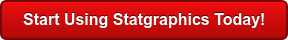# Data Mining & Machine Learning

Data Mining refers to a process by which patterns are extracted from data. Such patterns often provide insights into relationships that can be used to improve business decision making. Statistical data mining tools and techniques can be roughly grouped according to their use for clustering, classification, association, and prediction.Procedure Statgraphics Centurion 18 Statgraphics
Sigma express
Statgraphics
stratus
Statgraphics
Web Services
StatBeans
ClusteringClassificationAssociationPredictionClassification and Regression TreesText Mining#### Clustering

Clustering refers to data mining tools and techniques by which a set of cases are placed into natural groupings based upon their measured characteristics. Since the number of characteristics is often large, a multivariate measure of similarity between cases needs to be employed. When looking for how to data mine, Statgraphics provides a number of methods for deriving clusters, including nearest neighbor, furthest neighbor, centroid, median, group average, Ward's method, and the method of K-Means. The results may be displayed as a dendrogram, a membership table, or an icicle plot. Agglomeration plots are used to suggest the proper number of clusters.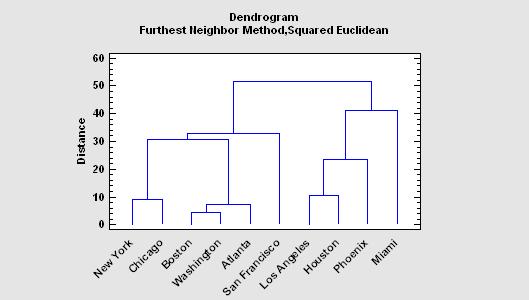More: Cluster Analysis.pdf

#### Classification

Classification is among the data mining tools and techniques by which a set of cases are assigned to levels of a categorical factor based upon their characteristics. A training set of known cases is used to develop a classification algorithm which can then be used to predict which category unknown cases are most likely to belong to. For example, applicants for a loan might be placed into risk categories based upon their personal characteristics, given an algorithm developed from previous applicants.
The Neural Network Classifier in Statgraphics uses a method based on nonparametric density function estimates combined with Bayesian priors.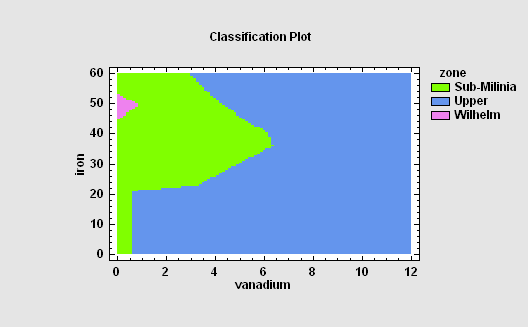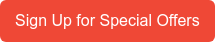#### Association

Measures of Association are used to identify variables that are related to each other. If the factors are quantitative, correlation coefficients may be used for statistical data mining tools and techniques like this. If the factors are non-quantitative, other measures of association are used for considering how to data mine. A matrix plot with nonlinear Lowess smoothers is shown below.

Statgraphics includes statistics such as Pearson's product-moment correlation coefficient, Kenkall and Spearman rank correlations, partial correlations, lambda, the uncertainty coefficient, Somer's D, the contingency coefficient, eta, Cramer's V, conditional gamma, Pearson's R, and Kendall's tau.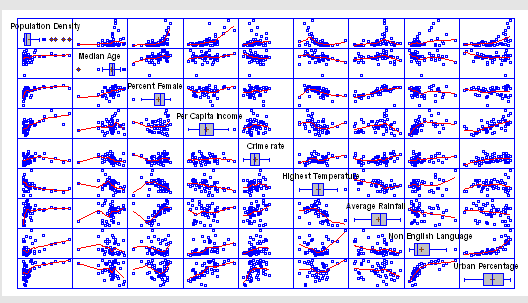#### Prediction

Prediction refers to the development of statistical models that can predict the value of one variable given the values of other variables. Regression models of various sorts are often used among data mining tools and techniques. When the number of predictors is large, selection of a good model can be difficult. In Statgraphics, the Regression Model Selection procedure of statistical data mining fits models involving all possible linear combinations of a set of predictors all selects the best models using criteria such as Mallows' Cp and the adjusted R-squared statistic.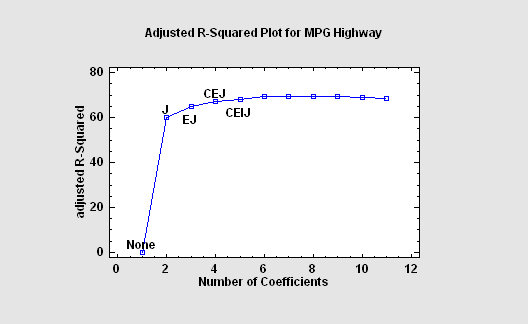#### Classification and Regression Tress

The Classification and Regression Trees procedure implements a machine-learning process to predict observations from data. It creates models of 2 forms:

1. Classification models that divide observations into groups based on their observed characteristics.
1. Regression models that predict the value of a dependent variable.

The models are constructed by creating a tree, each node of which corresponds to a binary decision. Given a particular observation, one travels down the branches of the tree until a terminating leaf is found. Each leaf of the tree is associated with a predicted class or value.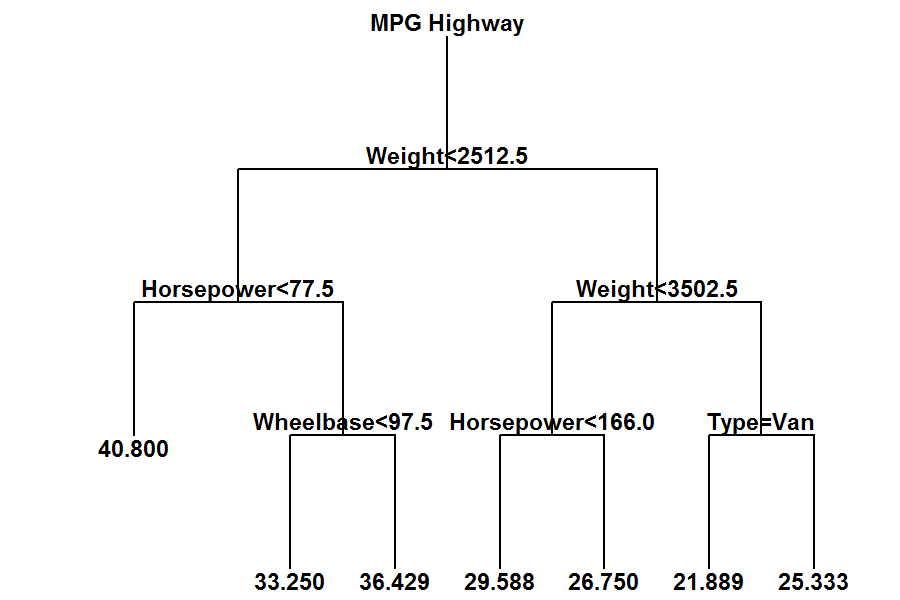#### Text Mining

The Text Mining procedure analyzes one or more text columns or documents to determine how frequently various words are used. The main output of this procedure is an identification of those words that occur most frequently. Both tabular and graphical summaries are provided.More: Text Mining.pdf or Watch Video